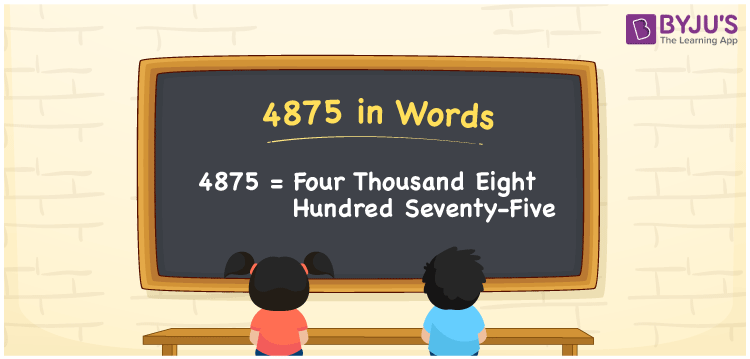# 4875 in Words

We can write 4875 in words as Four Thousand Eight Hundred Seventy-five. Suppose you sewing machine bought a new fan that costs Rs. 4875, then you could say that “ I bought a sewing machine for Four Thousand Eight Hundred Seventy-five rupees”. In this article, you will learn how to convert the number 4875 into words in an exciting way. The number 4875 is used in expressions that relate to money, days, distance, length, weight and so on.

 4875 in words Four Thousand Eight Hundred Seventy-five Four Thousand Eight Hundred Seventy-five in Numbers 4875

## 4875 in English Words

We generally write numbers in words using the English alphabet. Thus, we can read 4875 in English as “Four Thousand Eight Hundred Seventy-five”.## How to Write 4875 in Words?

In this section, you will learn how to write the number 4875 in words. The given number 4875 has four digits, so we should make a place value chart that represents the place value for all these four digits. The order of place values of digits in a number is in accordance with the Indian numbering system, such as:

• Ones
• Tens
• Hundreds
• Thousands
• Ten-thousands
• Hundred-thousands or Lakhs

This can be done as follows.

 Thousands Hundreds Tens Ones 4 8 7 5

Thus, we can write the expanded form as:

4 × Thousand + 8 × Hundred + 7 × Ten + 5 × One

= 4 × 1000 + 8 × 100 + 7 × 10 + 5 × 1

= 4875

= One thousand five hundred

4875 is a natural number that precedes 4874 and succeeds 4876.

4875 in words – Four Thousand Eight Hundred Seventy-five

Is 4875 an odd number? – No

Is 4875 an even number? – Yes

Is 4875 a perfect square number? – No

Is 4875 a perfect cube number? – No

Is 4875 a prime number? – No

Is 4875 a composite number? – Yes

## Frequently Asked Questions on 4875 in Words

### How do you write 4875 in English?

We can write 4875 in English words as “Four Thousand Eight Hundred Seventy-five”.

### How do you write 4875 in words for a cheque?

For a cheque, we generally write 4875 in words as Four Thousand Eight Hundred Seventy-five rupees only.

### What number is 4875 in words?

The number 4875 is Four Thousand Eight Hundred Seventy-five in words.